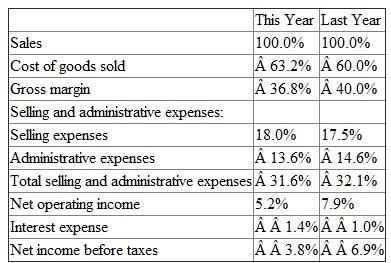# Quiz 15: Financial Statement Analysis

Business

1) Determine the common size percentage by dividing each line item by sales. Accordingly, Sales will always be 100%:2) Gross income has reduced dramatically, which is a result of the increase of cost of goods sold from 60.0% of sales last year to 63.2% of sales this year. It does not seem that the company is passing the increases in the costs of its products on to its customers. Selling expenses and interest expense also increased slightly during the year, which probably means that costs are generally going up in the company. The only exception is the administrative expenses, which the company could have made efforts to reduce during the year.

Horizontal analysis : Horizontal analysis examines how a particular item on a financial statement such as sales or cost of goods sold behaves over time. Vertical analysis: Vertical analysis involves analysis of items on an income statement or balance sheet for a single period. In vertical analysis of the income statement, all items are typically stated as a percentage of sales. In vertical analysis of the balance sheet, all items are typically stated as a percentage of total assets.

1)Gross margin percentage can be calculated as:2)Earnings per share can be calculated as:3)Price-earnings ratio can be calculated as:4)Dividend payout ratio can be calculated as:5)Dividend yield ratio can be calculated as:6)Return on total assets can be calculated as:7)Return on common stockholder's equity can be calculated as:8)Book value per share may be calculated as: